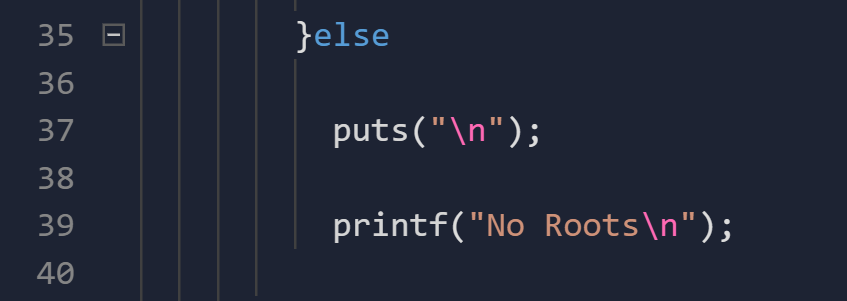← Back to all posts
##### I cannot understand why my ELSE is running with the IF

// LANGUAGE //

#include <stdio.h>
#include <math.h>

int main(void)
{

double a, b, c, delta, x, y;

``````  puts("put the value of a");

scanf("%lf",&a);

puts("put the value of b");

scanf("%lf",&b);

puts("put the value of c");

scanf("%lf",&c);

puts("\n");

delta = (b*b) - (4*a*c);

if (delta >= 0){

x = (-b + sqrt(delta))/(2*a);

y = (-b - sqrt(delta))/(2*a);

printf("Roots's Results: %.2lf e %.2lf", x, y);

puts("\n");

}else

puts("\n");

printf("No Roots\n");

return 0;``````

}

``     ``
Answered by Coder100 (18922) [earned 5 cycles]``````else {